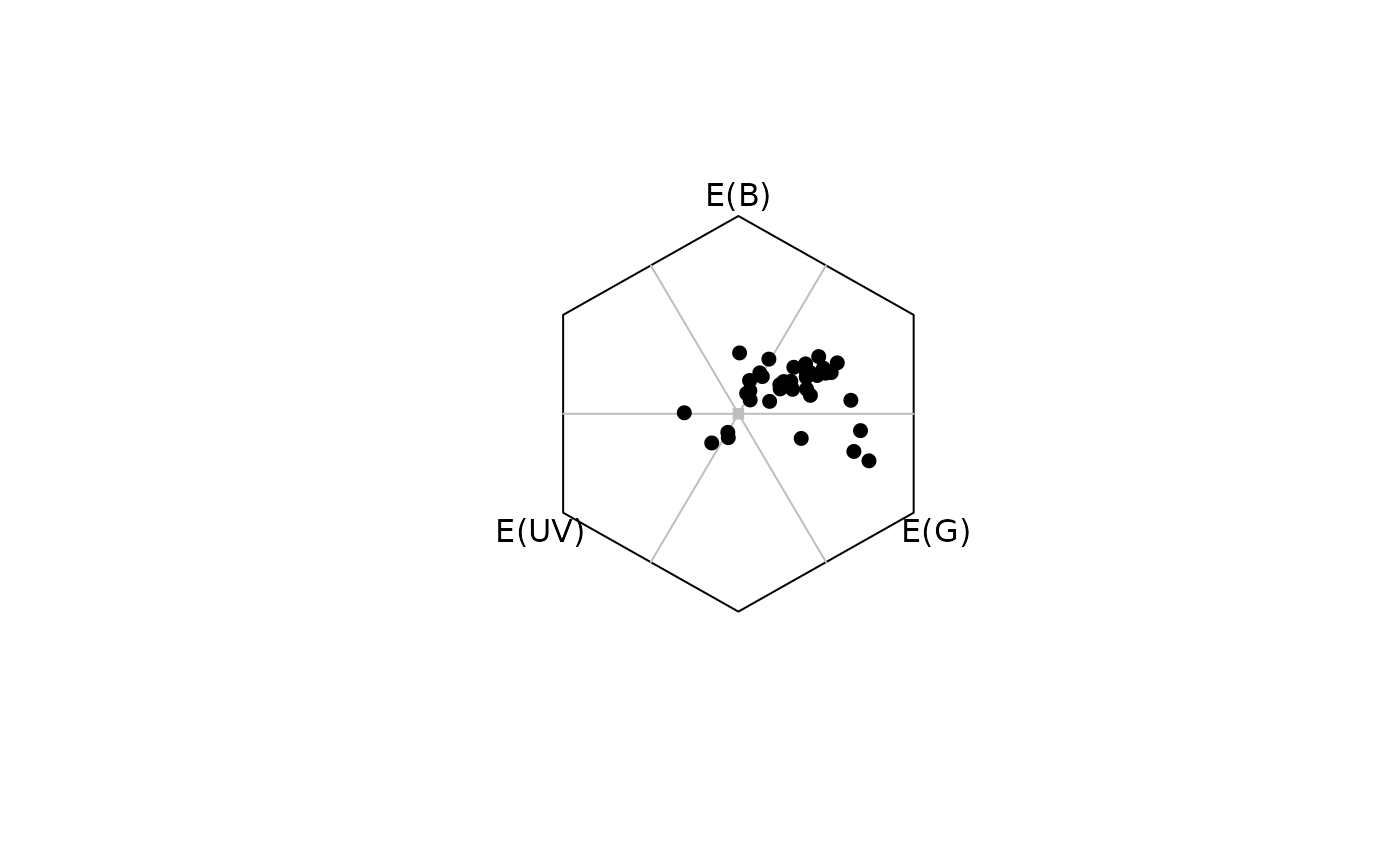Plots reflectance spectra in the appropriate colourspace.

# S3 method for colspace
plot(x, ...)

## Arguments

x

(required) an object of class colspace.

...

additional graphical options, which vary by modeled space. Refer to their individual documentation:

• diplot(): dichromat space

• triplot(): trichromat space

• tetraplot(): tetrahedral space

• catplot(): categorical space

• hexplot(): colour hexagon

• cocplot(): colour-opponent-coding space

• cieplot(): cie spaces

• segplot(): segment analysis space

• jndplot(): perceptual, 'noise corrected' space (for the results of jnd2xyz())

Also see par().

## Value

A colourspace plot appropriate to the input data.

plot(), points.colspace()

## Author

Rafael Maia rm72@zips.uakron.edu

Thomas White thomas.white026@gmail.com

## Examples

data(flowers)
data(sicalis)

# Dichromat
vis.flowers <- vismodel(flowers, visual = "canis")
di.flowers <- colspace(vis.flowers, space = "di")
plot(di.flowers)# Colour hexagon
vis.flowers <- vismodel(flowers,
visual = "apis", qcatch = "Ei", relative = FALSE,
vonkries = TRUE, achromatic = "l", bkg = "green"
)
hex.flowers <- colspace(vis.flowers, space = "hexagon")
plot(hex.flowers, sectors = "coarse")# Tetrahedron (static)
vis.sicalis <- vismodel(sicalis, visual = "avg.uv")
tcs.sicalis <- colspace(vis.sicalis, space = "tcs")
plot(tcs.sicalis)# Tetrahedron (interactive)
vis.sicalis <- vismodel(sicalis, visual = "avg.uv")
tcs.sicalis <- colspace(vis.sicalis, space = "tcs")
tcsplot(tcs.sicalis, size = 0.005)

## Add points to interactive tetrahedron
patch <- rep(c("C", "T", "B"), 7)
tcs.crown <- subset(tcs.sicalis, "C")
tcs.breast <- subset(tcs.sicalis, "B")
tcsplot(tcs.crown, col = "blue")
tcspoints(tcs.breast, col = "red")
3D plot